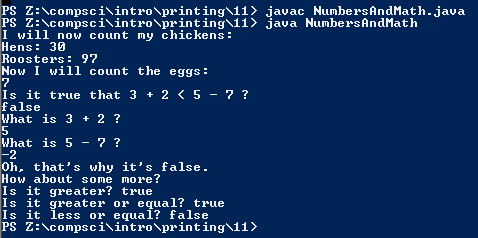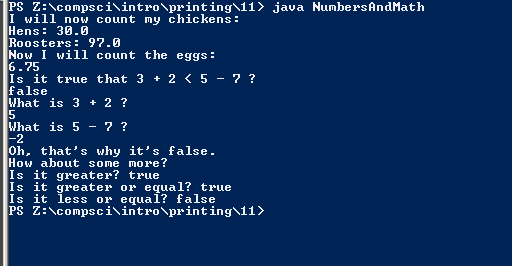# Assignment #11: Numbers and Math

## Code (No floating point numbers)

```    /// Name: Kiran O'Farrell
/// Period: 7
/// Program name: Numbers and Math
/// File Name: FirstProg.java
/// Date Finished: 9/2/15

public class NumbersAndMath {

public static void main(String[] args) {

System.out.println("I will now count my chickens:");

//This line divides 30 by 6, then adds the result to 25.
System.out.println("Hens: " + (25 + 30 / 6));

//This line multiplies 25 by 3, takes the remainder when that number is divided by 4, and then subtracts that number from 100.
System.out.println("Roosters: " + (100 - 25 * 3 % 4));

System.out.println("Now I will count the eggs:");

//This line takes the modulus of 4 and 2, divides 1 by 4, then adds 3, 2, 1, and 6 and subtracts those numbers and an additional 5 from the total. The number is rounded to the nearest integer.
System.out.println(3 + 2 + 1 - 5 + 4 % 2 - 1 / 4 + 6);

System.out.println("Is it true that 3 + 2 < 5 - 7 ?");

//This line checks to see whether the expression in the parentheses is true.
System.out.println(3 + 2 < 5 - 7);

System.out.println("What is 3 + 2 ?");

//This line adds 3 to 2 without comparing it to other results.
System.out.println(3 + 2);

System.out.println("What is 5 - 7 ?");

//This line does the same for 5 - 7.
System.out.println(5 - 7);

System.out.println("Oh, that's why it's false.");

//These lines are comparators like line 28.
System.out.println("Is it greater? " + (5 > -2));

System.out.println("Is it greater or equal? " + (5 >= -2));

System.out.println("Is it less or equal? " + (5 <= -2));
}
}
```

## Code (Edited to include floating point numbers)

```
//This line divides 30 by 6, then adds the result to 25.
System.out.println("Hens: " + (25.0 + 30.0 / 6.0));

//This line multiplies 25 by 3, takes the remainder when that number is divided by 4, and then subtracts that number from 100.
System.out.println("Roosters: " + (100.0 - 25.0 * 3.0 % 4.0));

System.out.println("Now I will count the eggs:");

//This line takes the modulus of 4 and 2, divides 1 by 4, then adds 3, 2, 1, and 6 and subtracts those numbers and an additional 5 from the total. The number is rounded to the nearest integer.
System.out.println(3.0 + 2.0 + 1.0 - 5.0 + 4.0 % 2.0 - 1.0 / 4.0 + 6.0);

```

### Picture of the output (No floating point numbers)### Picture of the output (With floating point numbers)